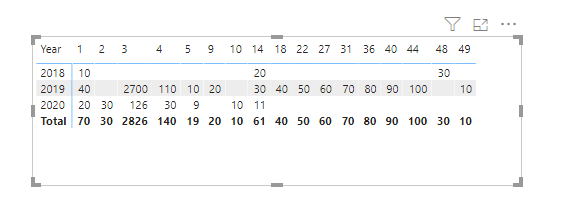cancel
Showing results for
Did you mean:New Member

## Create Weeks and calculate evolution

Hello everyone 🙂

Hope you're doing great!

I've got an issue: I've got a table with the number of sales during years (2017, 2018, 2019, 2020) aggregated by day.

However, I want to create a measure to create the dimension "Week", and being able to calculate the difference between a week to another.

For instance, Week 3 in 2020 is the week from 13th of January to 19th of January, and Week 3 in 2019 is 14th of January to 20th of January. I want to get able to calculate the evolution between Week 3 2019 to Week 2020.

thanks a LOT !!!

7 REPLIES 7Community Support

Hi @matrin ,

If you've fixed the issue on your own please kindly share your solution. If the above posts help, please kindly mark it as a solution to help others find it more quickly. Thanks!

Best Regards,
Yingjie LiCommunity Support

Hi @matrin ,

You can create these calculated columns to calculate each year week total sales:

``Week = WEEKNUM('Table'[Date],1)``
``````Total =
CALCULATE (
SUM ( 'Table'[Sales] ),
ALLEXCEPT ( 'Table', 'Table'[Week], 'Table'[Date].[Year] )
)``````

If you want to see the evolution, use a line chart and multi select the slicer with year and month:Here is my sample file that hopes to help you, please check and try it: Create Weeks and calculate evolution.pbix

Best Regards,
Yingjie Li

If this post helps then please consider Accept it as the solution to help the other members find it more quickly.could share the file again the link Create weeks and calculate evolution.pbix is brokenNew Member

This is exactly what I need, but I need to see evolutions (metrics) by comparing week 2 of 2020 and week 2 of 2019 in a table.... Do you think it is possible? @v-yingjlCommunity Support

Hi @matrin ,

Did you mean use the Matrix to show it? I don't think it can relfect better than a line chart though the matrix needn't use the slicer.Best Regards,
Yingjie Li

If this post helps then please consider Accept it as the solution to help the other members find it more quickly.New Member

Thanks a lot for your help!

Can't I just calculate the evolution thanks to a new metric? I need to add the evolution in my table, that is whySuper User

@matrin , You can weekrank and week number for that to compare Same week 364 day behind or 52 week behind. Have date table with following columns

Week Start date = 'Date'[Date]+-1*WEEKDAY('Date'[Date],2)+1
Week End date = 'Date'[Date]+ 7-1*WEEKDAY('Date'[Date],2)
Week Number = WEEKNUM([Date],2)
Week = if('Date'[Week Number]<10,'Date'[Year]*10 & 'Date'[Week Number],'Date'[Year]&'Date'[Week Number])
Week Rank = RANKX(all('Date'),'Date'[Week Start date],,ASC,Dense)

This Week = CALCULATE(sum('order'[Qty]), FILTER(ALL('Date'),'Date'[Week Rank]=max('Date'[Week Rank])))
Last Week = CALCULATE(sum('order'[Qty]), FILTER(ALL('Date'),'Date'[Week Rank]=max('Date'[Week Rank])-1))

Last year Week = CALCULATE(sum('order'[Qty]), FILTER(ALL('Date'),'Date'[Week Rank]=max('Date'[Week Rank])-52))

Week Year behind Sales = CALCULATE(SUM(Sales[Sales Amount]),dateadd('Date'[Date],-364,DAY))

To get the best of the time intelligence function. Make sure you have a date calendar and it has been marked as the date in model view. Also, join it with the date column of your fact/s. Refer :
https://www.archerpoint.com/blog/Posts/creating-date-table-power-bi
https://www.sqlbi.com/articles/creating-a-simple-date-table-in-dax/